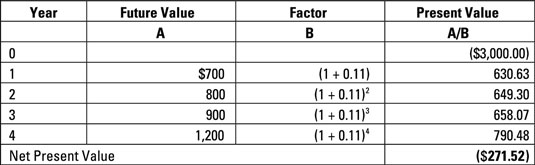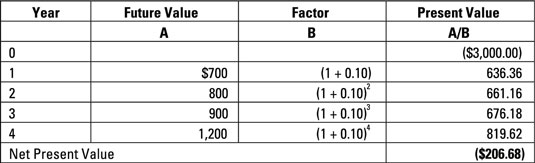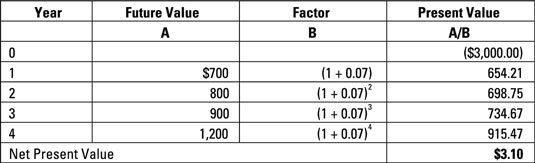##### Managerial Accounting For DummiesWhen evaluating a capital project, internal rate of return (IRR) measures the estimated percentage return from the project. It uses the initial cost of the project and estimates of the future cash flows to figure out the interest rate. In general, companies should accept projects with IRR that exceed the cost of capital and reject projects that don’t meet that guideline.

Using the NPV method, you can figure out internal rate of return through trial and error — plug different interest rates into your formulas until you figure out which interest rate delivers an NPV closest to zero.

Consider Corporation X’s proposed project costing \$3,000. Managers project positive net cash inflow of \$700 one year from now, \$800 in two years, \$900 in three years, and \$1,200 in four years. An interest rate of 11 percent yields an NPV of –\$271.52.Recompute the NPV, using a lower interest rate such as 10 percent.This rate results in an NPV of –\$206.68. No good. Try a much lower interest rate, like 7 percent.The extremely low net present value of \$3.10 for this experiment indicates that the internal rate of return for this project is about 7 percent.

Computing internal rate of return may require estimating the NPV for several different interest rates and estimating an interest rate to one-tenth of 1 percent, judging which rate results in the lowest NPV. Microsoft Excel offers powerful functions for computing internal return of return, as do many financial calculators.

Granted, using trial and error to compute IRR may be frustrating. This process can be all the more daunting because IRR usually leads managers to make the same decision as NPV does. Any project with positive NPV will also have IRR that exceeds the cost of capital.

However, NPV values are difficult to compare across different projects. Naturally, a large project (with a large investment) should have a higher NPV than a smaller project (with a smaller investment). However, IRR takes into account the size of the investment, allowing you compare different-sized projects alongside each other.